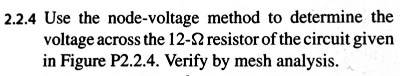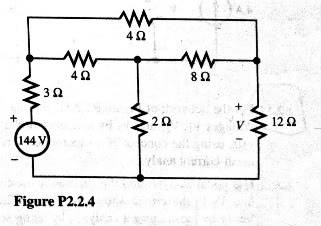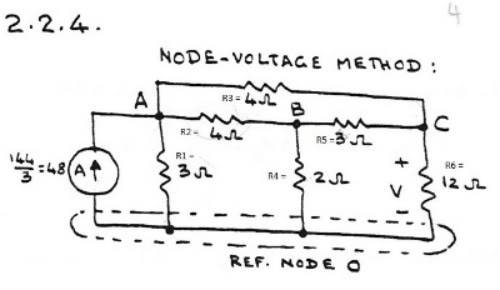# Circuits: Solve Using Nodal and mesh Analysis

In summary, the conversation discusses applying KCL and KVL to a circuit with resistors R1, R2, and R3. The author considers whether the resistors are in parallel and writes node voltage equations for each node A, B, and C. The conversation ends with encouragement to continue with mesh analysis.

## Homework StatementKCL & KVL:

## The Attempt at a Solution

Ok folksI am not sure where to go from here. Someone suggested changing the 144V to its Thevenin equivalent, which I have done below. I know that I am supposed to apply KCL at each node A,B, and C. And then I represent each current in terms of voltages. Now I am a little confused as to how to proceed.

I am trying got convince myself that R1, R2, and R3 are in parallel because their leads are all connected, but I am not sure yet. The voltage drop across R1 is definitely just VA since I can pass from A across the resistor and directly to ground. But for R2 and R3, I feel like the voltages at B and C need to be considered.

Any thoughts?I think you meant to say that you've changed the voltage supply and its series resistance to its Norton equivalent.

Also, one leg in common does not a parallel connection make; R1, R2, and R3 are not in parallel.

With the network as shown in your second diagram, you are in a position to write the node voltage equations by inspection.

gneill said:
I think you meant to say that you've changed the voltage supply and its series resistance to its Norton equivalent.

Also, one leg in common does not a parallel connection make; R1, R2, and R3 are not in parallel.

With the network as shown in your second diagram, you are in a position to write the node voltage equations by inspection.

So they are not in parallel because they do not share the same voltage, correct?

I think I have it now:

Node A:

$48 - V_A/3 - (V_B - V_A)/4 - (V_C - V_A)/4 = 0$

Node B:

$(V_B - V_A)/4 - (V_C - V_B)/3 - V_B/2 = 0$

Node C:

$-V_C/12 + (V_C - V_B)/3 + (V_C - V_A)/4 = 0$

Now I need to move forward to the mesh analysis.

For components to be in parallel, both ends of each need to be directly connected to each other.

It looks like you're on the right track. Keep up the good work!